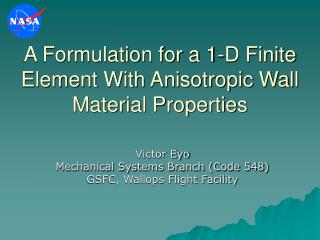DownloadDownload PresentationA Formulation for a 1-D Finite Element With Anisotropic Wall Material Properties

# A Formulation for a 1-D Finite Element With Anisotropic Wall Material Properties

Télécharger la présentation## A Formulation for a 1-D Finite Element With Anisotropic Wall Material Properties

- - - - - - - - - - - - - - - - - - - - - - - - - - - E N D - - - - - - - - - - - - - - - - - - - - - - - - - - -
##### Presentation Transcript

1. A Formulation for a 1-D Finite Element With Anisotropic Wall Material Properties Victor Eyo Mechanical Systems Branch (Code 548) GSFC, Wallops Flight Facility

2. Motivation • Old college assignment • Lack of 1-Dimensional finite element capable of anisotropic material property input • Possible research tool for balloon structural analyses • Closed form solution eliminates singularities encountered with traditional shell elements FEMCI 2006 Oct 25 & 26FEMCI 2006 Oct 25 & 26

3. z Vy My r L q Vx Mx T P Geometry & Loading • Geometry Considered • Thin walled cantilevered cylindrical tubes • Length, L • Radius r • Generalized coordinate, (r,q) pair • Loading • Loaded at free end via some kind of reinforcement system • Shear loads, Vx & Vy • Moments, Mx & My • Torque, T • Axial load, P FEMCI 2006 Oct 25 & 26FEMCI 2006 Oct 25 & 26

4. Constitutive Equation • Assumptions • Wall bending stiffness neglected • Shape of cross-section maintained during deformation • Reinforcement system (spar, web,…) for proper load distribution • Resulting Analysis Model • Circumferential strains disappear • Wall considered membrane in plane stress • State of stress at any point on the wall can be expressed as FEMCI 2006 Oct 25 & 26FEMCI 2006 Oct 25 & 26

5. q N q dq q q dz N z q Constitutive Equation • In terms of stress resultant • N: “tension flow” • q: shear flow FEMCI 2006 Oct 25 & 26FEMCI 2006 Oct 25 & 26

6. Constitutive Equation • Inverting i(,z): elastic constants, in general will vary with  and z, but assumed constant here FEMCI 2006 Oct 25 & 26FEMCI 2006 Oct 25 & 26

7. The n-Ply Symmetric Laminate • Laminate Stress Strain Relationship 2 q 1 Qij(k) : In-plane global stiffness matrix FEMCI 2006 Oct 25 & 26FEMCI 2006 Oct 25 & 26

8. The n-Ply Symmetric Laminate • Expand • Solve • Compare FEMCI 2006 Oct 25 & 26FEMCI 2006 Oct 25 & 26

9. w(q,z) Y(z) v(q,z) q (z) X(z) r Wall Displacement • In-plane • Displacements • Rotations • Small displacement theory • Out of plane • Displacements & rotations assumed to be of form Assume k=1 for this work FEMCI 2006 Oct 25 & 26FEMCI 2006 Oct 25 & 26

10. Stress-Displacement Relationship • Linearized stress-displacement relationship • Ref: Reissner, E., On the Variational Theorem of Finite Elastic Deformations, Journal of Mathematics and Physics, 32;129-135, July 1953 • Libove, Charles, On the Stiffness, Stresses and Buckling Analysis of Corrugated Shear Webs, In Wei-Wen Yu (Editor), Second Specialty Conference on Cold Formed Steel Structures: Current Research and Design Trends, pages 259-302, October 1973 FEMCI 2006 Oct 25 & 26FEMCI 2006 Oct 25 & 26

11. Displacements & Twist • Substitute for u(q,z) • Extract differential equations & natural boundary conditions • Derive Total Potential Energy () of beam system • Minimize the TPE • requires its first variation =0 • Solve DEQs applying prescribed boundary conditions FEMCI 2006 Oct 25 & 26FEMCI 2006 Oct 25 & 26

12. z Vy My r L q Vx Mx T Displacements & Twist FEMCI 2006 Oct 25 & 26FEMCI 2006 Oct 25 & 26 P

13. NASTRAN Model • Wall: QUAD4 Elements • 586 nodes; 574 Elements • z axis used for orientation reference • Membrane and bending coupling • Capped Free end: QUAD4 with high stiffness • RBE3 rigid elements used to simulate displacements at section mid-node FEMCI 2006 Oct 25 & 26FEMCI 2006 Oct 25 & 26

14. Results • Tube Geometry • r=0.8in • t=0.04in • L=14.0in • Tube wall • AL2024-T4 • Isotropic • Homogenous • Layered Composite Laminates • E-Glass/Epoxy [(0/±45/90)]s • T300/5208 Gr/E [(+20/-70)2]s • Loading • Vx=Vy=10.0lb • Mx=My=60.0in-lb • T=123.0in-lb • P=480.0lb FEMCI 2006 Oct 25 & 26FEMCI 2006 Oct 25 & 26

15. A22=0.476 A23=0.0 A33=0.160 A11=0.476 A12=0.157 A13=0.0 Results –AL2024-T4 1=0.424 2=0.0 4=0.159 FEMCI 2006 Oct 25 & 26FEMCI 2006 Oct 25 & 26

16. A22=0.476 A23=0.0 A33=0.160 A11=0.476 A12=0.157 A13=0.0 Results – AL2024-T4 1=0.424 2=0.0 4=0.159 FEMCI 2006 Oct 25 & 26FEMCI 2006 Oct 25 & 26

17. A22=0.476 A23=0.0 A33=0.160 A11=0.476 A12=0.157 A13=0.0 Results – AL2024-T4 1=0.424 2=0.0 4=0.159 FEMCI 2006 Oct 25 & 26FEMCI 2006 Oct 25 & 26

18. A22=0.233 A23=0.0 A33=0.090 A11=0.233 A12=0.054 A13=0.0 Results – E-Glass/Epoxy 1=0.221 2=0.0 4=0.090 FEMCI 2006 Oct 25 & 26FEMCI 2006 Oct 25 & 26

19. A22=0.233 A23=0.0 A33=0.090 A11=0.233 A12=0.054 A13=0.0 Results – E-Glass/Epoxy 1=0.221 2=0.0 4=0.090 r=51.5% FEMCI 2006 Oct 25 & 26FEMCI 2006 Oct 25 & 26

20. A22=0.233 A23=0.0 A33=0.090 A11=0.233 A12=0.054 A13=0.0 Results – E-Glass/Epoxy r=5.7% 1=0.221 2=0.0 4=0.090 FEMCI 2006 Oct 25 & 26FEMCI 2006 Oct 25 & 26

21. A22=0.408 A23=-0.102 A33=0.155 A11=0.408 A12=0.107 A13=0.102 Results – T300/5208 1=0.380 2=-0.129 4=0.089 FEMCI 2006 Oct 25 & 26FEMCI 2006 Oct 25 & 26

22. A22=0.408 A23=-0.102 A33=0.155 A11=0.408 A12=0.107 A13=0.102 Results – T300/5208 1=0.380 2=-0.129 4=0.089 FEMCI 2006 Oct 25 & 26FEMCI 2006 Oct 25 & 26

23. A22=0.408 A23=-0.102 A33=0.155 A11=0.408 A12=0.107 A13=0.102 Results – T300/5208 1=0.380 2=-0.129 4=0.089 FEMCI 2006 Oct 25 & 26FEMCI 2006 Oct 25 & 26

24. Conclusion • Work in progress – no major conclusions • Possible path to developing a 1-D finite element with anisotropic material properties FEMCI 2006 Oct 25 & 26FEMCI 2006 Oct 25 & 26